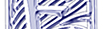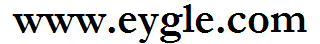首页 技术基础 备份恢复 SQL优化 诊断案例 BLOG 留言板 服务 生活 网摘 阅读 下载 墨天轮 English

### Oracle HowTo:如何使用Leading提示改变表连接方式The `LEADING` hint causes Oracle to use the specified table as the first table in the join order.

If you specify two or more `LEADING` hints on different tables, then all of them are ignored. If you specify the `ORDERED` hint, then it overrides all `LEADING` hints.

 `SQL> SELECT /*+ leading(t_max) use_hash(t_max t_middle) */ COUNT (*)  2    FROM t_small, t_max, t_middle  3  WHERE t_small.object_id = t_middle.object_id  4  AND t_middle.object_id = t_max.object_id  5  /` `Execution Plan----------------------------------------------------------   0      SELECT STATEMENT Optimizer=CHOOSE (Cost=262 Card=1 Bytes=12)   1    0   SORT (AGGREGATE)   2    1     HASH JOIN (Cost=262 Card=400 Bytes=4800)   3    2       HASH JOIN (Cost=225 Card=113776 Bytes=910208)   4    3         TABLE ACCESS (FULL) OF 'T_MAX' (Cost=151 Card=113792 Bytes=455168)   5    3         TABLE ACCESS (FULL) OF 'T_MIDDLE' (Cost=39 Card=28447 Bytes=113788)   6    2       TABLE ACCESS (FULL) OF 'T_SMALL' (Cost=2 Card=100 Bytes=400) `

 `SQL> SELECT /*+ ordered use_hash(t_max t_middle) */ COUNT (*)  2    FROM  t_max, t_middle,t_small  3  WHERE t_small.object_id = t_middle.object_id  4  AND t_middle.object_id = t_max.object_id  5  /` `Execution Plan----------------------------------------------------------   0      SELECT STATEMENT Optimizer=CHOOSE (Cost=228 Card=1 Bytes=12)   1    0   SORT (AGGREGATE)   2    1     HASH JOIN (Cost=228 Card=400 Bytes=4800)   3    2       TABLE ACCESS (FULL) OF 'T_SMALL' (Cost=2 Card=100 Bytes=400)   4    2       HASH JOIN (Cost=225 Card=113776 Bytes=910208)   5    4         TABLE ACCESS (FULL) OF 'T_MAX' (Cost=151 Card=113792 Bytes=455168)   6    4         TABLE ACCESS (FULL) OF 'T_MIDDLE' (Cost=39 Card=28447 Bytes=113788)`

>> 2011-02-26文章:
>> 2009-02-26文章:
>> 2005-02-26文章:

By eygle on 2006-02-26 12:21 | Comments (5) | HowTo | SQL.PLSQL | 694 |

SQL> SELECT /*+ ordered use_hash(t_max t_middle) use_hash(t_middle,t_small) */ COUNT (*)
2 FROM t_max, t_middle ,t_small
3 WHERE t_small.object_id = t_middle.object_id
4 AND t_middle.object_id = t_max.object_id
5 /

Execution Plan
----------------------------------------------------------
0 SELECT STATEMENT Optimizer=CHOOSE (Cost=33 Card=1 Bytes=39)
1 0 SORT (AGGREGATE)
2 1 HASH JOIN (Cost=33 Card=50 Bytes=1950)
3 2 TABLE ACCESS (FULL) OF 'T_SMALL' (Cost=2 Card=50 Bytes
4 2 HASH JOIN (Cost=30 Card=5000 Bytes=130000)
5 4 TABLE ACCESS (FULL) OF 'T_MAX' (Cost=6 Card=29561 By
6 4 TABLE ACCESS (FULL) OF 'T_MIDDLE' (Cost=2 Card=5000

zf_wu,是的,对于多表(3表以上)的连接,很难严格控制连接方式.